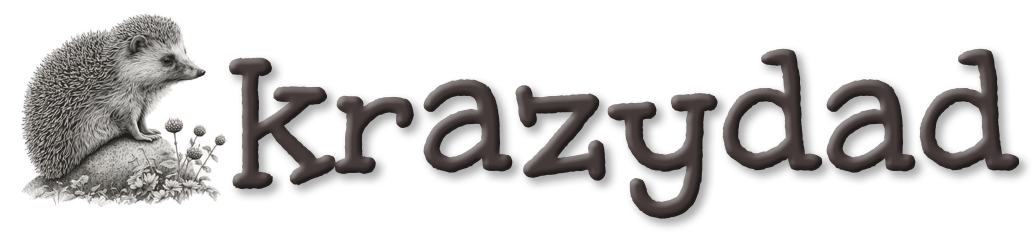Blog  |   Puzzles  |   Books  |   About

# Double Rainbow All The Way

An HTML5/canvas tutorial by Jim Bumgardner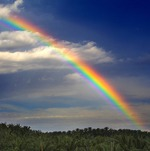In this tutorial, we're going to make an animated double rainbow in HTML5 / Canvas. I'm doing this to teach you about the HSL color space, and to show you a more sophisticated way of using it.

There is also a version of this tutorial with the same examples written for Processing.js or Processing. There are tradeoffs using either system, but for the most part, it's a bit easier in Processing. Note that if you are using an ancient and terrible web browser (IE8 or earlier), you won't be able to see the examples!

To draw a rainbow, we need a graphics framework to work with. In this tutorial, I'm going to draw everything using the Canvas APIs which are part of HTML5. This means that if you are using an older web browser, like IE 8 or earlier, you're not going to see anything!

All the examples I'm using make use of the same basic skeleton code, which sets up a simple canvas and draws in it using its device context. You can download this skeleton, here, and use it for your own experiments.

To draw in the canvas, I will place the code in a routine called refresh, like so.

```function refresh(dc, width, height)
{
}
```

The refresh routine accepts as parameters the device context (dc) of the canvas, the width of the canvas, and the height of the canvas. Click on my examples to see the contents of my refresh() function.

For the occasional animated example, I will use a slightly modified skeleton that also passes in a frame number, like so: (You'll find an additional piece of sample code for doing animations in the above archive).

```function refresh(dc, width, height, frame_number)
{
}
```

Although I have supplied you with the skeleton code, you'll learn more if you attempt to build it from scratch yourself, using an HTML5 canvas reference.

We'll start by making a rectangular bar, which is colored like a rainbow. To do this, I'm going to draw a series of lines of different colors. I'll start by just coloring them randomly, using the random number generator.

If you click on Example 1, you can see the code. If you refresh the page, the bar will look different because the random number generator produces different numbers each time. It doesn't look much like a rainbow, but it's something to start with. I've created four useful utility functions. The first simplifies getting integer random numbers:

```function myRandom(n)
{
return Math.floor( Math.random() * n );
}
```
The second utility function creates a CSS rgb color string:
```function rgbColor(r,g,b)
{
return 'rgb(' + r + ',' + g + ',' + b + ')';
}
```
You may be familiar with specifying colors in CSS using a specification like '#FF7F00', in which the R, G and B components are specified in hexadecimal. The rgb style allows you to get the same color using 3 decimal numbers (one for red, green, and blue respectively). So instead of using #FF7F00 you would say rgb(255,127,0). Since those CSS colors are supplied as strings, this function allows me to construct it from individual numbers.

The third utility function uses myRandom to produce a random color:

```
function randomRGBColor()
{
return rgbColor(myRandom(256),myRandom(256),myRandom(256));
}
```

Here we combine the previous two functions in a useful way; supplying the R,G and B values with random numbers. Each color component has a range of 256 values, so I'm generating a random number from 0 to 255. The last utility function is used to draw a line in a particular color:

```function line(dc,color,x1,y1,x2,y2)
{
dc.strokeStyle = color;    // set the color
dc.beginPath();            // create the path
dc.moveTo(x1,y1);
dc.lineTo(x2,y2);
dc.stroke();               // stroke along the path
}
```
This is pretty straight-forward. It is passed a device context (dc), a CSS color string, and the line coordinates. It sets the color, creates a path for the line, and then strokes the color along the path.

Finally, we get to the part of the code that draws the bar. The bar is made up of 1-pixel lines. First I set up the coordinates of the top left edge of the bar, which are specified by left_margin and top_margin. Then I set the width and height of the bar. Finally, I draw each line, from top to bottom, in a for-loop. As the for-loop executes, the variable i takes on the values 0,1,2,3... all way to the height of the bar. This variable is used to set the Y position for each line.

```var left_margin = 10;
var top_margin = 10;
var w = width - left_margin*2;
var h = height - top_margin*2;

for (var i = 0; i < h; ++i) {
line(dc, randomRGBColor(), left_margin, top_margin+i, left_margin+w, top_margin+i);
}
```
Now, in a rainbow, the colors aren't random, so let's take the randomness out. I'll change the loop so that the colors go from black to red.

Here I've removed the random color function, and changed the loop, to read as follows:

```for (var i = 0; i < h; ++i) {
var ratio = i/h;
var red = Math.floor(256*ratio);
var green = 0;
var blue = 0;
line(dc, rgbColor(red,green,blue), left_margin, top_margin+i, left_margin+w, top_margin+i);
}
```
By the way, if I were writing this for myself, the interior of the loop would be a single line, without all the temporary variables:
```for (var i = 0; i < h; ++i) {
line(dc, rgbColor( Math.floor(256*i/h), 0, 0), left_margin, top_margin+i, left_margin+w, top_margin+i);
}
```
The temporary variables are there to help illustrate what is going on. The variable ratio is i/h. During the loop it takes on values from 0 to just below 1. I can then multiply this ratio by any other number to produce numbers in any range I like.

The red color is produced by multiplying the ratio by 256, and then using Math.floor() to convert it to an integer. Since the ratio never quite hits 1, the color value will never hit 256 (which is good, because the RGB color components are supposed to go from 0 to 255).

Now I'll modify the code so as the red goes from 0 up to 255, the green goes in the other direction, from 255 down to 0.

Here I've changed the line that sets the green:

```  var green = Math.floor(256 * (1-ratio) );
```
By using 1-ratio, I make the value go backwards, from 1 to almost 0.

I could probably combine a bunch of these gradient bars and produce a pretty good looking rainbow effect, but the code would be longer than necessary - there would need to be a separate loop for each band. Fortunately, there's an easier way. Instead of using a CSS rgb() string, I'll use a CSS3 hsl() string. This looks like this:

hsl( hue, saturation%, luminance%)

Hue is a degree value that goes from 0 to 360. It starts red, then goes through all the colors of the rainbow, and then comes back to red. Saturation is a percentage value from 0 to 100% which tells how colorful the color is (from grayscale at 0, to fully saturated at 100%). Luminance is a percentage value from 0 to 100% which tells how bright the color is (from black at 0, to white at 100%). Note that in this color model, extremely dark and extremly light colors aren't very saturated. If you use 100% for luminance, the color will look white. To get extremely bright and saturated colors, use 50% luminance. Here's a function to make hsl() strings from numbers, similar to our rgbColor() function.
```function hslColor(h,s,l)
{
return 'hsl(' + h + ',' + s + '%,' + l + '%)';
}
```
And here's a gradient which uses a ratio to walk through the available hues, drawing the brightest colors possible. Things are starting to look a little rainbowy, no?

The main drawing loop (which again could be reduced to one inner line if you were so inclined) reads as follows:

```for (var i = 0; i < h; ++i) {
var ratio = i/h;
var hue = Math.floor(360*ratio);
var sat = 100;
var lum = 50;
line(dc, hslColor(hue,sat,lum), left_margin, top_margin+i, left_margin+w, top_margin+i);
}
```

Many programmers would be happy with this gradient for a rainbow effect, and might stop here, which is fine. Let's take a break, and when we come back, we'll make it look even more like a rainbow.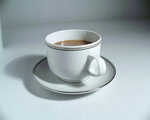Since I'll soon be adding some translucency, I'd like to get some sky behind the rainbow. This can be done by painting a sky-blue rectangle behind the rainbow. I googled to find some reasonable values for sky blue, and came up with some code, like so:

```var skyBlue = '#87CEEB';
dc.fillStyle = skyBlue;
dc.fillRect(0,0,width,height);
```
And here's what it looks like. I made the rainbow bar a little smaller than it was in example 4, so you can see the blue background behind it better.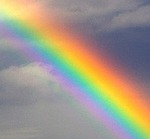It's tempting to just use this linear hue sweep to produce a rainbow, but I'd like to make it a little more true to nature. If you search for photographs of rainbows, you'll notice that the colors appear a little different than the fake rainbow in example 5.

Notice how in the photograph, the yellow stripe is nearly in the middle, whereas in example 5, the yellow stripe happens much closer to the top. Also notice how near the top of the real rainbow, the colors change more slowly and at the bottom they seem to change faster. It takes about the same time to get from red to yellow (which are close in hue) as it does to get from yellow to magenta, which are far apart in hue.

We can approximate this effect by multiplying our ratio by itself (or squaring it). It will still go from 0 to 1, because 0*0 = 0 and 1*1 = 1, but when it would normally be at .5, it will be at .5 * .5 or .25.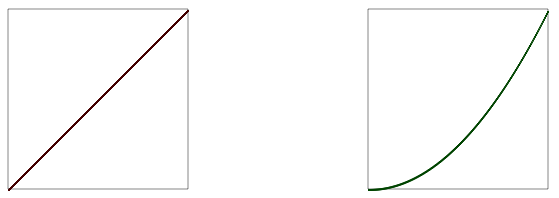Graphically this makes the slope of parameter look like the green line (a power curve) instead of like the red line (linear or flat). Another way of describing the green curve is "ease in". The colors in a real rainbow ease in. This slope more closely resembles the change in hue of a real rainbow and you can see the result in example 6:

All we've done is change the line:
```  var hue = Math.floor(360*ratio);
```
to
```  var hue = Math.floor(360*ratio*ratio);
```
It still isn't an exact match, but it's much better. Next, the rainbow will look better if it is translucent, especially on the edges. It turns out there is an CSS hsla() color specification that allows you to add a number to indicate opacity or "alpha". By tweaking the alpha, we get example 7. I used half of a sine wave for the alpha so that it would rise and fall as we draw across the bar. Take a look at the code of example 7 to see how I did it.

Now let's change those lines to curves, and we'll get the familiar rainbow arch.

This is accomplished by changing our line function to an arch function, and changing our loop to draw each line of the arch.
```function arch(dc,color,cx,cy,rad)
{
dc.strokeStyle = color;
dc.beginPath();
dc.arc(cx, cy, rad, Math.PI, 2*Math.PI, false);
dc.stroke();
}

var cx = width/2;
var cy = height;
var rainbowThick = width/10;

dc.lineWidth = 2;

for (var i = 0; i < rainbowThick; ++i) {
var ratio = i/rainbowThick;
var hue = Math.floor(360*ratio*ratio);
var sat = 100;
var lum = 50;
var alpha = Math.sin(Math.PI*ratio) * 0.75;
}
```

When I first did this, it produced some white pixels (moire artifacts) because the curves didn't line up exactly. I fixed this by doubling the line width of the pen. Let's take a break, we've accomplished a lot!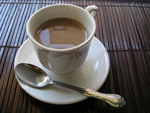Now let's do some more work on the background. It would be nice to have a gradient in the sky, just like we have in the rainbow. We could draw a bunch of lines again, but there is an easier way -- we can use the HTML5/Canvas gradient functions.

Here's the code:

```var skyBlue = '#87CEEB';
var lightSkyBlue = '#87CEFA';
var deepSkyBlue = '#00BFFF';

dc.fillRect(0,0,width,height
```

So let's make a double rainbow, shall we? Looking at photos of double rainbows (and the small handful of double rainbows I have seen in my life time), I know that the outer rainbow is dimmer, and that the colors travel in the opposite direction. Here I've taken a stab at the general effect.

Finally, let's add a little animation, by cycling the saturation, and the luminance.

I've also added some foreground fog to this final version. Hmm, it would be awesome if there were some puffy clouds to go with the rainbow. I guess that'll have to wait for the next tutorial. Have fun!

Oh, in case you haven't seen it, here's my tutorial on drawing circles, spirals and sunflowers.

If you are in the Los Angeles area, I'll be teaching a couple of graphics and music programming workshops in Culver City, using the Processing language, in the next few weeks. More info here.